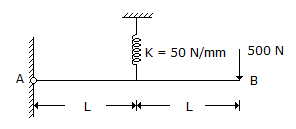# Civil Engineering - UPSC Civil Service Exam Questions

11.

Which one of the following is not essential to derive the formula used in constructing a synthetic unit hydrograph ?

 A. Time to peak B. peakflow C. Inter flow D. Time lease

Explanation:

No answer description available for this question. Let us discuss.

12.

In an aerial puhotogrammetric survey, if the exposure interval is 20 seconds to cover ground distance of 1000 m between exposures, what would be the ground speed of the aircraft ?

 A. 90 km/hour B. 120 km/hour C. 150 km/hour D. 180 km/hour

Explanation:

No answer description available for this question. Let us discuss.

13.

The head over a 90° V-notch weir increases from 0.15 m to 0.3 m. The ratio of new discharge to the original discharge is, nearly equal to

 A. 5.65 B. 1.42 C. 4 D. 2.62

Explanation:

No answer description available for this question. Let us discuss.

14.

Consider the following statements with ragard to crack formation and its control:
1. The surface width of the crack should not, in general, exceed 0.30 mm for structures not subjected to aggressive environment.
2. When depth of web in a beam exceeds 750 mm, side face reinforcement @ 0.1 percent of web area should be provided on each face.
3. The nominal spacing of main bars in a slab should not exceed three times the effective depth of a solid slab or 300 mm, whichever is smaller.
Which of the statements given above is/are correct ?

 A. 1 only B. 1 and 2 C. 1 and 3 D. 2 and 3

Explanation:

No answer description available for this question. Let us discuss.

15.

A rigid bar is supported by a spring as shown in the given figure.The deflection of the point B will be

 A. 10 mm upward B. 20 mm downward C. 5 mm upward D. 40 mm downward.

Explanation:

The Force in spring F = (500x2L)/L = 1000N.

Deflection in the spring δ = F/k = 1000/50 = 20mm.

Deflection at the point B = 2δ = 40mm downward.